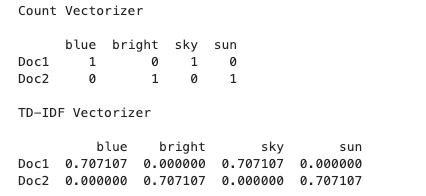# TF-IDF Vectorizer scikit-learn

`Train Document Set:d1: The sky is blue.d2: The sun is bright.Test Document Set:d3: The sun in the sky is bright.d4: We can see the shining sun, the bright sun.`
`# TfidfVectorizer # CountVectorizerfrom sklearn.feature_extraction.text import TfidfVectorizer,CountVectorizerimport pandas as pd# set of documentstrain = ['The sky is blue.','The sun is bright.']test = ['The sun in the sky is bright', 'We can see the shining sun, the bright sun.']# instantiate the vectorizer objectcountvectorizer = CountVectorizer(analyzer= 'word', stop_words='english')tfidfvectorizer = TfidfVectorizer(analyzer='word',stop_words= 'english')# convert th documents into a matrixcount_wm = countvectorizer.fit_transform(train)tfidf_wm = tfidfvectorizer.fit_transform(train)#retrieve the terms found in the corpora# if we take same parameters on both Classes(CountVectorizer and TfidfVectorizer) , it will give same output of get_feature_names() methods)#count_tokens = tfidfvectorizer.get_feature_names() # no differencecount_tokens = countvectorizer.get_feature_names()tfidf_tokens = tfidfvectorizer.get_feature_names()df_countvect = pd.DataFrame(data = count_wm.toarray(),index = ['Doc1','Doc2'],columns = count_tokens)df_tfidfvect = pd.DataFrame(data = tfidf_wm.toarray(),index = ['Doc1','Doc2'],columns = tfidf_tokens)print("Count Vectorizer\n")print(df_countvect)print("\nTD-IDF Vectorizer\n")print(df_tfidfvect)`spicy sparse matrix of count and tf-idf vectorizer
`Train Document Set:d1: The sky is blue.d2: The sun is bright.Test Document Set:d3: The sun in the sky is bright.d4: We can see the shining sun, the bright sun.`
`#import count vectorize and tfidf vectorisefrom sklearn.feature_extraction.text import CountVectorizer, TfidfVectorizertrain = ('The sky is blue.','The sun is bright.')test = ('The sun in the sky is bright', 'We can see the shining sun, the bright sun.')# instantiate the vectorizer object# use analyzer is word and stop_words is english which are responsible for remove stop words and create word vocabularycountvectorizer = CountVectorizer(analyzer='word' , stop_words='english')terms = countvectorizer.fit_transform(train)term_vectors  = countvectorizer.transform(test)print("Sparse Matrix form of test data : \n")print(term_vectors.todense())`
`idf vector= (2.09861229 1. 1.40546511 1.)matrix form of idf =[[2.09,0,0,0], [0,1,0,0], [0,0,1.40,0], [0,0,0,1]]`
`# Tranfer  sparse matrix of Countvectorizer to tf-idf by # using TfidfTransformerfrom sklearn.feature_extraction.text import TfidfTransformertfidf = TfidfTransformer(norm='l2')term_vectors.todense()#[0, 1, 1, 1]# [0, 1, 0, 2]tfidf.fit(term_vectors)tf_idf_matrix = tfidf.transform(term_vectors)print("\nVector of idf \n")print(tfidf.idf_)print("\nFinal tf-idf vectorizer matrix form :\n")print(tf_idf_matrix.todense())`
`from sklearn.feature_extraction.text import TfidfVectorizertrain = ('The sky is blue.','The sun is bright.')test = ('The sun in the sky is bright', 'We can see the shining sun, the bright sun.')# instantiate the vectorizer object# use analyzer is word and stop_words is english which are responsible for remove stop words and create word vocabularytfidfvectorizer = TfidfVectorizer(analyzer='word' , stop_words='english',)tfidfvectorizer.fit(train)tfidf_train = tfidfvectorizer.transform(train)tfidf_term_vectors  = tfidfvectorizer.transform(test)print("Sparse Matrix form of test data : \n")tfidf_term_vectors.todense()`
`References :For Photo and Pictures:https://machinelearningflashcards.com/`

--

--